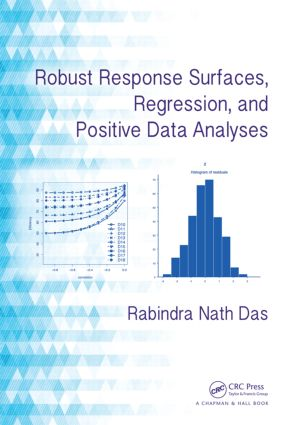# Robust Response Surfaces, Regression, and Positive Data Analyses

## 1st Edition

Chapman and Hall/CRC

336 pages | 45 B/W Illus.

##### Purchasing Options:\$ = USD
Hardback: 9781466506770
pub: 2014-05-21
\$125.00
x
eBook (VitalSource) : 9780429088667
pub: 2014-05-21
from \$28.98

FREE Standard Shipping!

### Description

Although widely used in science and technology for experimental data generating, modeling, and optimization, the response surface methodology (RSM) has many limitations. Showing how robust response surface methodology (RRSM) can overcome these limitations, Robust Response Surfaces, Regression, and Positive Data Analyses presents RRS designs, along with the relevant regression and positive data analysis techniques. It explains how to use RRSM in experimental designs and regression analysis.

The book addresses problems of RRS designs, such as rotatability, slope-rotatability, weak rotatability, and optimality. It describes methods for estimating model parameters as well as positive data analysis techniques. The author illustrates the concepts and methods with real examples of lifetime responses, resistivity, replicated measures, and more.

The range of topics and applications gives the book broad appeal both to theoreticians and practicing professionals. The book helps quality engineers, scientists in any area, medical practitioners, demographers, economists, and statisticians understand the theory and applications of RRSM. It can also be used in a second course on the design of experiments.

### Reviews

"… this book stands [as the] first of its kind … The author explains well the utilization of RRS methods in experimental designs and regression analysis. The author ingeniously furthers the explanation on the problem of RRS designs … This book elucidates these methods to both theoreticians and researchers besides practical users, like engineers in quality control, … demographers, hydro-geologists, economists, statisticians, biological and physical scientists, and environmental and agricultural scientists …"

Zentralblatt MATH 1306

INTRODUCTION

The Problem and the Perspective

A Brief Review of the Literature

Scope of the Present Textbook

Robust Rotatable Designs

Summary of the Work Done

Concluding Remarks

ROBUST FIRST-ORDER DESIGNS

Introduction and Summary

First-Order Correlated Model

Robust First-Order Designs

Robust First-Order Designs for Intra-Class Structure

Robust First-Order Designs for Inter-Class Structure

Robust First-Order Designs for Generalized Inter-Class Structure

Robust First-Order Designs for Compound Symmetry Structure

Robust First-Order Designs for Tri-Diagonal Structure

Robust First-Order Designs for Auto Correlated Structure

Concluding Remarks

ROBUST SECOND-ORDER DESIGNS

Introduction and Summary

Second-Order Correlated Model

Robust Second-Order Rotatability

Robust Second-Order Designs for Intra-Class Structure

Robust Second-Order Designs for Inter-Class Structure

Robust Second-Order Designs for Compound Symmetry Structure

Robust Second-Order Designs for Tri-Diagonal Structure

Robust Second-Order Designs for Auto Correlated Structure

Concluding Remarks

LIFETIME IMPROVEMENT ROBUST FIRST-ORDER DESIGNS

Introduction and Summary

Location-Scale Lifetime First-Order Correlated Model

Lifetime Improvement (LI) Robust First-Order Designs

LI Robust First-Order Designs for Intra-Class Structure

LI Robust First-Order Designs for Inter-Class Structure

LI Robust First-Order Designs for Compound Symmetry Structure

LI Robust First-Order Designs for Tri-Diagonal Structure

LI Robust First-Order Designs for Autocorrelated Structure

Concluding Remarks

LIFETIME IMPROVEMENT ROBUST SECOND-ORDER DESIGNS

Introduction and Summary

Location-Scale Lifetime Second-Order Correlated Model

Lifetime Improvement Robust Second-Order Rotatability

LI Robust Second-Order Designs for Intra-Class Structure

LI Robust Second-Order Designs for Inter-Class Structure

LI Robust Second-Order Designs for Compound Symmetry Structure

LI Robust Second-Order Designs for Tri-Diagonal Structure

LIRobust Second-Order Designs for Autocorrelated Structure

Concluding Remarks

WEAKLY ROBUST ROTATABLE DESIGNS

Introduction and Summary

Deviation from Rotatability

Weakly Robust First-Order Rotatable Designs

Weakly Robust Second-Order Rotatable Designs

Concluding Remarks

Appendix

ROBUST SECOND-ORDER SLOPE-ROTATABILITY

Introduction and Summary

Robust Second-Order Slope-Rotatability Conditions

Robust Second-Order Slope-Rotatable Designs

Modified Robust Second-Order Slope-Rotatability Conditions

Robust Second-Order Slope-Rotatable Designs for Intra-Class Structure

Robust Second-Order Modified Slope-Rotatable Designs for Intra-Class Structure

Illustration

Concluding Remarks

ROBUST SECOND-ORDER SLOPE-ROTATABILITY OVER ALL DIRECTIONS

Introduction and Summary

Estimation of Derivatives

Robust Second-Order Slope-Rotatability over All Directions

Robust Second-Order Slope-Rotatable Designs over All Directions for

Concluding Remarks

ROBUST SECOND-ORDER SLOPE-ROTATABLE DESIGNS WITH MAXIMUM DIRECTIONAL VARIANCE AND D-OPTIMAL SLOPE

Introduction and Summary

Robust Balanced Designs

Robust Second-Order Slope-Rotatability with Maximum Directional Variance

Robust Second-Order Slope-Rotatable Designs with Maximum Directional Variance

D-Optimal Robust Second-Order Slope-Rotatability

D-Optimal Robust Second-Order Slope-Rotatability Conditions

D-Optimal Robust Second-Order Slope-Rotatable Designs

Conel Uding Remarks

WEAKLY ROBUST SECOND-ORDER SLOPE-ROTATABLE DESIGNS

Introduction and Summary

Robust Second-Order Slope-Rotatability Measures

Illustrations

Concluding Remarks

REGRESSION ANALYSIS FOR CORRELATED OBSERVATIONS

Introduction and Summary

Regression Model with Correlated Errors

Estimation of Regression Parameters for

Inference on Regression Parameters for

Regression Diagnostics with Correlated Errors

Index of Fit

Illustration

Location-Scale Lifetime Regression Model

Estimation of Regression Parameters for Location-Scale Lifetime Model with

Illustration

Concluding Remarks

APPLICATIONS OF CORRELATED REGRESSION ANALYSES

Introduction and Summary

Analysis of a Randomized Block Design with Correlated Errors

A Reinforced Randomized Block Design with Correlated Errors

POSITIVE DATA ANALYSES

Introduction and Summary

Log-Normal and Gamma Model with Constant Variance

Log-Normal and Gamma Model with Non-Constant Variance

Discrepancy in Regression Estimate between the Two Models for Constant Variance

Discrepancy in Regression Estimates between the Two Models for Non-Constant Variance

Discrepancy in Fitting between the Two Models for Non-Constant Variance

Concluding Remarks

APPLICATIONS OF LOG-NORMAL AND GAMMA MODELS

Introduction and Summary

Lifetime Data Analysis Based on Log-Normal Model

Resistivity Data Analysis Based on Log-Normal and Gamma Model

Multiple Responses Data Analysis Based on Gamma Model

Human Biochemical Parameters Relationships

Active Factors on Human Body Mass Index

The Role of Iodine in the Thyroid Status of Mother and Her Neonates

Effects of Wine Drinking on Liver Biochemical Parameters

Risk Factors of Diabetes Mellitus to Pima India Mothers

Personal Characteristics and Dietary Impacts on Plasma Concentrations

Infant Mortality in India

Concluding Remarks

### Subject Categories

##### BISAC Subject Codes/Headings:
MAT029000
MATHEMATICS / Probability & Statistics / General
TEC032000
TECHNOLOGY & ENGINEERING / Quality Control